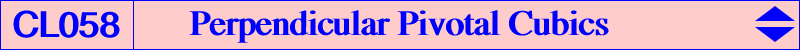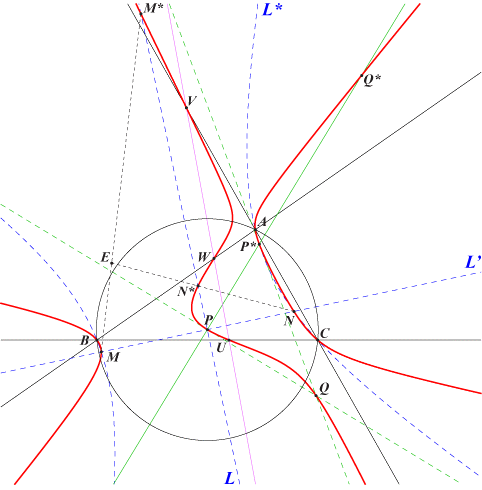Let P be a fixed finite point and an isoconjugation with pole Ω. Let L be a variable line through P and let L' be its perpendicular at P. The isoconjugate L* of L intersects L' at two points M, N. When L varies, the locus of M, N is a circumcubic we shall provisionally denote by K(Ω, P) and call the perpendicular pivotal cubic with pole Ω and pivot P. This cubic can be seen as the locus of point X such that the circle with diameter XX* passes through P where X* is the isoconjugate of X*. Obviously, L and L* intersect at two points on pK(Ω, P). L intersects the isoconjugate of L' at two points which are the isoconjugates M*, N* of M, N. Thus we have the four collinearities on the cubic : P, M, N – P, M*, N* – P*, M, N* – P*, M*, N. Theorem : K(Ω, P) is the nK with pole Ω, root ocP (the orthocorrespondent of P), passing through P and P*. Other points on the cubic : • U, V, W on the sidelines of ABC. These are the traces of the orthotransversal of P. • A' = PA /\ P*U, B' and C' similarly. • U' = PU /\ P*A, V' and W' similarly. • the perpendicular at P to PP* meets the circumconic passing through P, P* at a second point Q on the cubic. Q is actually the common tangential of P and P*. • Q* is the third point of the cubic on the line PP*. The points AU /\ A'U', BV /\ B'V', CW /\ C'W' are collinear and lie on a fixed line passing through Q* and E, the intersection of the lines MM* and NN*. • The five points Q, M, N, M*, N* lie on a conic passing through the third point R of the cubic on the line QQ*. R* is the common tangential of Q and Q*.For a given pivot P, all the cubics K(Ω, P) belong to a same net of cubics generated by three decomposed cubics. One of them is the union of the lines AB, AC, PU and the two other are obtained similarly. Each cubic contains A, B, C, P, U, V, W. *** Special cubics of the net Circular cubics For any pivot P that is not the orthocenter H, the net contains one and only one circular cubic with pole P x igP, the barycentric product of P and the inverse (in the circumcircle) of the isogonal conjugate of P. When P = H, the pole must lie on the orthic axis but, in this case, the cubic decomposes into the line at infinity and a rectangular circum-hyperbola. Theorem : with P ≠ H, nK(P x igP, ocP, P) is a focal cubic with singular focus igP. The cubic meets the circumcircle (O) again at the trilinear pole T of the line passing through the Lemoine point K and the pole P x igP. Its real point at infinity is the isoconjugate of T. Note that the pole cannot be K when P ≠ H. This focal cubic can be seen as the locus of point X such that the circle with diameter XX* passes through P where X* is the isoconjugate of X in the isoconjugation that swaps P and igP. When P = X(316), the Droussent pivot, the pole is the centroid G of ABC. This gives K091, the only isotomic focal cubic. More generally, if Ω ≠ K is given then P is the reflection of the trilinear pole of KΩ about the Ω-isoconjugate of (O) i.e. the trilinear polar of tgΩ (isotomic conjugate of the isogonal conjugate of Ω). The following table gives a selection of these focal cubics according to their foci F.F centers on the cubic Ω root P X(1) X(1), X(80), X(522), X(2222) X(2161) X(2006) X(80) X(2) X(2), X(67), X(525), X(935) X(67) ? X(67) X(4) X(4), X(265), X(476), X(523) X(1989) ? X(265) X(6) X(6), X(523), X(671), X(691) X(111) ? X(671) X(32) X(32), X(512), X(805), X(1916) ? ? X(1916) X(54) X(54), X(523), X(1263), X(1291) ? ? X(1263) X(67) X(67), X(99), X(316), X(523) K091 X(2) ? X(316) X(186) X(3), X(110), X(186), X(526) X(50) X(1993) X(3) X(187) X(2), X(110), X(187), X(690) X(187) X(1992) X(2) X(468) X(69), X(99), X(468), X(690) X(524) ? X(69) X(1319) X(8), X(100), X(900), X(1319) X(44) X(1997) X(8) X(1326) X(10), X(101), X(1326), X(2786) ? X(1999) X(10) X(1691) X(76), X(99), X(804), X(1691) X(385) ? X(76)Equilateral cubics When P = H, K(Ω, P) is equilateral for Ω on the orthic axis but the cubic degenerates as already said. Let ∆ be the perpendicular bisector ∆ of OH. ∆ is the image of the circumcircle (O) in the isoconjugation with pole X(1989) i.e. in the isoconjugation that swaps the Fermat points X(13) and X(14). When P lies on ∆, there is a family of equilateral cubics K(Ω, P) and all of them have the same pole Ω = X(1989). K(X1989, X5) = nK(X1989, X1994, X5) is an example of such cubic and this contains X(5), X(1113), X(1114), X(1141). For any point P ≠ H not lying on ∆, there is one and only one equilateral cubic K(Ω, P). For example, with P = X(1), X(2), X(3), X(8) we obtain the cubics nK(X2161, X57, X1), nK(X115, X1992, X2), nK(X6, X1993, X3) = K105, nK(X37, X1997, X8) respectively.